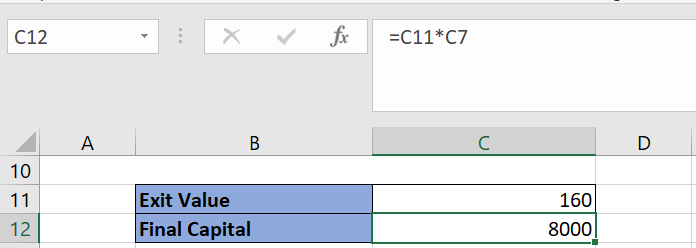# How to calculate Stop Loss in an Excel Sheet?

While designing trading systems, it is imperative to have a stop loss to prevent capital erosion. It helps traders to protect their capital in the event of high volatility and whipsaw signals. In this post, we are going to learn how to calculate stop loss in an excel sheet. The stop loss can be fixed or expressed in terms of the percentage of your equity.

## Fixed Stop Loss

A fixed stop loss is applied when you already know when to exit the trade in the cases when it goes against you. For example: if you buy a stock at 100, and you have predetermined that you need to exit when it goes below 95. So, your stop loss would be 5 points. A fixed stop loss can be determined based on support-resistance levels, pivot points or price action.

## Percentage Stop Loss

A percentage stop loss is expressed in terms of the percentage of your total equity. For example: if you invested 10000 for buying a stock, and you are willing to lose max 2% if the trade goes south. Then, you need to exit when the current equity value becomes 9800.

In general, the percentage stop loss is more prevalent among system traders as it is independent of security’s price.

## Calculate Stop Loss in Excel Sheet

In order to calculate stop loss in excel sheet, you will need to enter the following parameters:

1. Initial Capital: Your base equity using which you enter the trade.
2. Entry Value: The price of the security at the time of entering the trade.
3. Stop Loss Type: The type of stop loss as described above (fixed or percentage)
4. Stop Loss Value: The value of stop loss. If the stop loss type is fixed, it should be entered in terms of points. And if the stop loss type is percentage, it should be entered in terms of percentage.

Using the above input parameters, the below calculations are automatically performed in the excel sheet.

1. Number of Units: The total number of securities that can be purchased with your initial capital. It is calculated by dividing the initial capital with entry value.The floor function is used to eliminate fractions.

1. Exit Value: If the stop loss type is fixed, the exit value is simply the difference between entry value and stop loss value. And if the stop loss type is percentage, then x% of entry value is subtracted from the entry value to derive the exit value. Here “x” is the stop loss value.1. Final Capital: The final capital is exit value multiplied by the number of stocks.